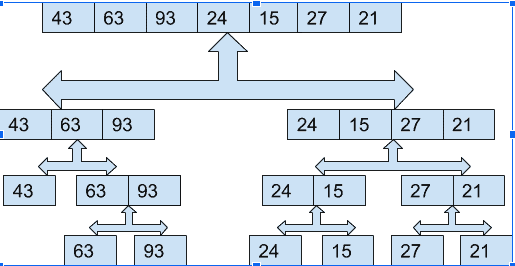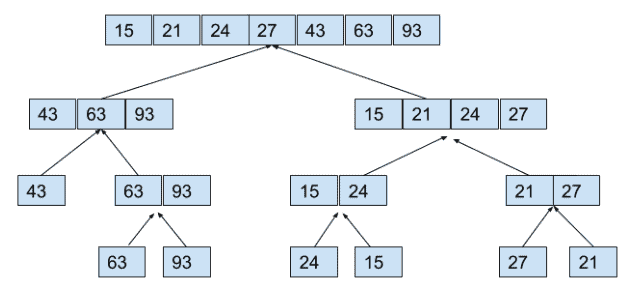# Merge Sort in Java: Everything You Should Know

Like QuickSort, the merge sort is a sorting method in which the array is divided into two halves, sorted by these halves. After sorting, these halves are merged. This process recursively occurs as every half of the array is again divided into two halves, sorted and merged.

## Merge Sort in Java

Java Merge sort is the “divide and conquers” algorithm in which we first divide the problem into many subproblems.  Then, it divides the input array into two halves, calls itself for them, and then merges those two sorted halves.

The merge() function is used for merging the two halves. So, in one sentence, we can say, in Merge Sort, we perform Divide Operation, then Conquer and Combine Operation.

When we have solutions for the subproblems, we combine them to get a final solution.

It is a straightforward algorithm, which can be easily implemented using the recursion as we deal with the subproblems rather than the big main problem.

In a nutshell, the MergeSort algorithm can be described as the following two-step process:

1. Divide: In this step, we divide an input array into 2 halves, the pivot being the midpoint of an array. This step is carried out recursively for all the half arrays until there are no more half arrays to divide.
2. Conquer: In this step, we sort and merge a divided array from a bottom to top and get the sorted array.

## Explanation with Example

The processing of elements in each pass can be understood as follows:

Assume that we are given the following array, which is to be sorted.

 43 63 93 24 15 27 21

Here, the size of the array is 7. So, we divide it into two halves. As the number of elements is odd here, our second half will have an element more than the first half.

 43 63 93 24 15 27 21

Now, these two halves will be sorted and merged. But this will again happen in the same manner by dividing into halves, sorting, and merging.

Hence the following algorithm will be followed.

```int[] Merge_sort(arr[], start, end)
if(end>start):
mid=(start+end)/2
first[]=Merge_sort(arr, start, mid)
second[]=Merge_sort(arr, mid+1, end)
arr[]=Merge( first, second)
return arr[]```
 43 63 93 24 15 27 21

So, in the above example, the sorting of the array will take place as follows.So, after dividing the array and separating them in a single unit, we will now perform conquer operation where this sorting will be served. Below, the diagram shows how this to conquer operation will be performed and the final sorted array.And finally, these two halves will be merged:

 15 21 24 27 43 63 93

## Java Program of Merge Sort

The code for Merge Sort in Java is the following.

```import java.util.*;

public class MergeSort {
public static void main(String[] args) {
Scanner sc = new Scanner(System.in);
System.out.println("Enter the size of the Array: ");
int size_of_arry = sc.nextInt(); // size of array, to b entered by the user
int arr[] = new int[size_of_arry];
System.out.println("Enter Array Elements ");
for (int i = 0; i < size_of_arry; i++)
arr[i] = sc.nextInt(); // element of array, to be entered by the user
arr = Merge_sort(arr, size_of_arry);
System.out.println("Array after Merge Sort is: ");
for (int i = 0; i < size_of_arry; i++)
System.out.print(arr[i] + " ");
System.out.println("\n");
}

static int[] Merge_sort(int arr[], int size) {
if (size > 1) {
int mid = size / 2;
int[] first = Arrays.copyOfRange(arr, 0, mid);
first = Merge_sort(first, mid); // recursive call for first half array
int[] second = Arrays.copyOfRange(arr, mid, size);
second = Merge_sort(second, size - mid); // recursive call for second half array
arr = Merge_arrays(first, second, mid, size - mid);
}
return arr;
}

static int[] Merge_arrays(int first[], int second[], int n, int m) // respectively
{
int arr[] = new int[n + m];
int i = 0, f = 0, s = 0;
while (f < n && s < m) {
if (first[f] < second[s])
arr[i++] = first[f++];
else
arr[i++] = second[s++];
}
while (f < n)
arr[i++] = first[f++];
while (s < m)
arr[i++] = second[s++];
return arr;
}
}
```

## Time Complexity:

The time complexity for the Merge Sort algorithm is O(n log n).

The time complexity for the program code remains the same in all cases, i.e., worst case or best case.

## Application:

1. Merge Sort is useful for sorting linked lists in O(n log n) time.
2. It is used in Inversion Count Problem.
3. We can use it in External Sorting.
4. If a lot of parallelization occurs, then the parallelizing Mergesort is more straightforward than other algorithms.
5. You can use the merge sort when you need the stable sort. It’s an essential feature of the merge sort.
6. When sorting the file which doesn’t fit into memory, you might break it into the chunks which fit into the memory, sort these using independently, writing each out to the file, then merge sort the generated files.

That’s it for this tutorial.

This site uses Akismet to reduce spam. Learn how your comment data is processed.